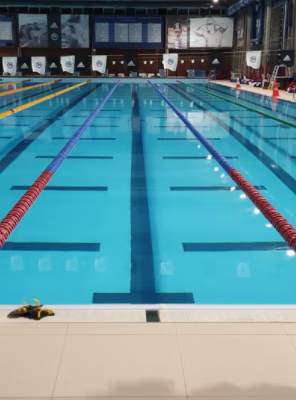## Swimming Pool Pressure

Water has a density of 997 kg/m^3. Logically, estimate the difference in pressure between the bottom and surface of a typical Olympic sized swimming pool located at sea level on Earth. Make any necessary assumptions.Hint
$$\Delta P=\rho gh$$$where $$\Delta P$$ is the pressure difference, $$\rho$$ is the density of water, $$g$$ is the acceleration due to gravity, and $$h$$ is the pool’s depth. Hint 2 Note, atmospheric pressure acts both on the pool’s bottom and surface, and should not factor into the pressure difference. Answers will vary. In our case, we will assume no atmospheric pressure difference between the pool’s surface and bottom, and a depth of 3 m. The pressure difference is: $$\Delta P=\rho gh$$$
where $$\Delta P$$ is the pressure difference, $$\rho$$ is the density of water, $$g$$ is the acceleration due to gravity, and $$h$$ is the pool’s depth. Thus,
$$\Delta P=(997\frac{kg}{m^3})(9.81\frac{m}{s^2})(3m)=29\: kPa$$\$
It is also acceptable to leave height (h) in the solution as a variable. Note, atmospheric pressure acts both on the pool’s bottom and surface, and should not factor into the pressure difference.
Answers will vary. In our case, we will assume no atmospheric pressure difference between the pool’s surface and bottom, and a depth of 3 m, which will give a 29 kPa pressure difference. It is also acceptable to leave height (h) in the solution as a variable.